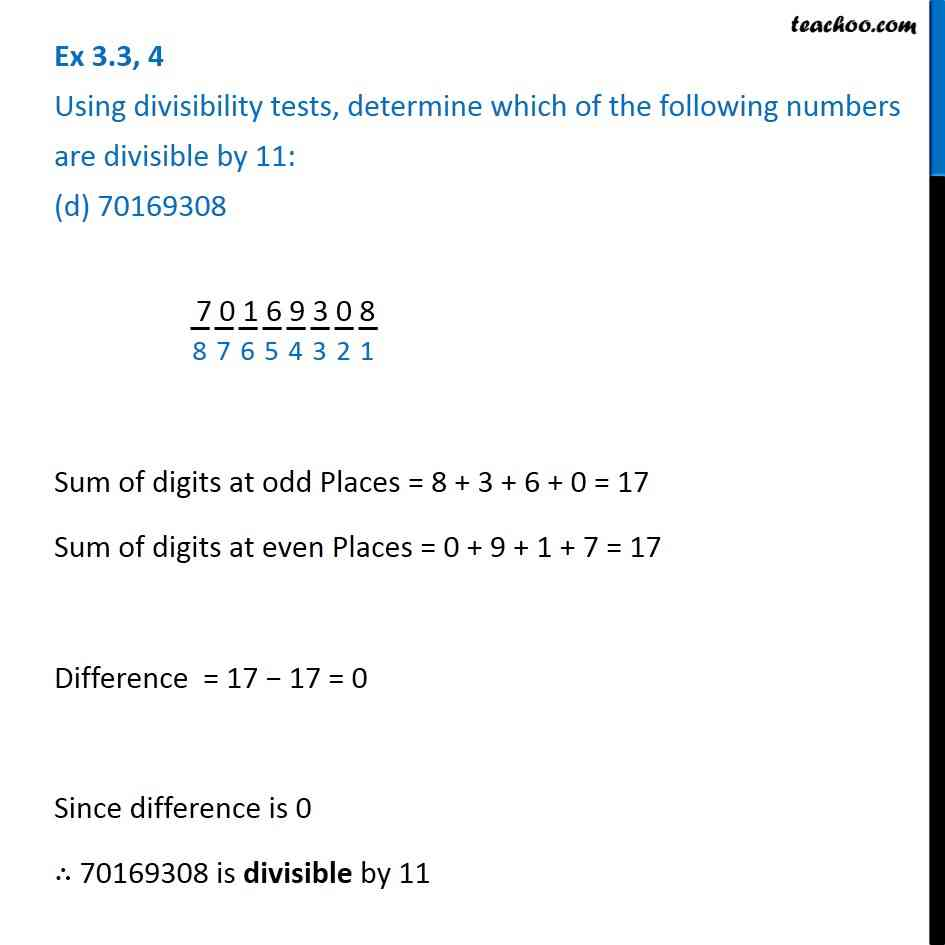Ex 3.3

Chapter 3 Class 6 Playing with Numbers
Serial order wiseLearn in your speed, with individual attention - Teachoo Maths 1-on-1 Class

### Transcript

Ex 3.3, 4 Using divisibility tests, determine which of the following numbers are divisible by 11: (d) 70169308 7 0 1 6 9 3 0 8 Sum of digits at odd Places = 8 + 3 + 6 + 0 = 17 Sum of digits at even Places = 0 + 9 + 1 + 7 = 17 Difference = 17 − 17 = 0 Since difference is 0 ∴ 70169308 is divisible by 11